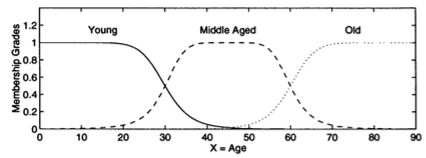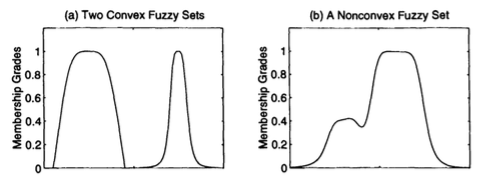### Linguistic Variables

In practice, when the universe of discourse X is a continuous space ( the real line R or its subset ), we usually partition X into several fuzzy sets whose MFs cover X in a more or less uniform manner. These fuzzy sets, which usually carry names that conform to adjectives appearing in our daily linguistic usage, such as "large", "medium" or "small" are called linguistic values or linguistic labels. Thus, the universe of discourse X is often called linguistic variable. Formal definitions of linguistic variables and linguistic value are given in this section. Here is a simple example.#### Linguistic variables and linguistic values

Suppose that X = "age". Then we can define fuzzy sets "young", "middle aged" and "old" that are characterized by MFs μyoung(x), μmiddleaged(x) and μold(x), respectively. Just as variable can assume various values, a linguistic variable "Age" can assume different linguistic values, such as "young", "middle aged" and "old" in this case. If "age" assumes the value of "young", then we have the expression "age is young", and so forth for the other values. Typical MFs for these linguistic values are displayed in the figure below, where the universe of discourse X is totally covered by the MFs and the transition from one MF to another is smooth and gradual.

A fuzzy set is unique specified by its membership function. To describe membership functions more specifically, the nomenclature are used in the literature.

#### Support

The support of a fuzzy set A is the set of all points x in X such that μA(x) > 0:

`support(A) = { x | μA(x) > 0 }`

#### Core

The core of a fuzzy set A is the set of all points x in X such that μA(x) > 1:

`core(A) = { x | μA(x) = 1 }`

#### Normality

A fuzzy set A is normal if its core is nonempty. In other words, we can always find a points x ∈ X such that μA(x) = 1

#### Crossover points

A crossover point of a fuzzy set A is a point x ∈ X at which μA(x) = 0.5

`crossover(A) = { x | μA(x) = 0.5 }`

#### Fuzzy singleton

A fuzzy set whose support is single point in X with x μA(x) = 1 is called a fuzzy singleton.

Figure below illustrate the cores, supports and crossover points of the bell-shaped membership function representing "middle aged" and of the fuzzy singleton characterizing "45 years old".#### α-cut and strong α-cut

The α-cut or α-level set of a fuzzy set A is a crisp set defined by

`Aα = { x | μA(x) ≥ α }`

Strong α-cut or strong α-level set are defined similarly:

`Aα' = { x | μA(x) > α }`

Using the notation for a level set, we can express the support and core of a fuzzy set A as

`support(A) = A0'`

and

`core(A) = A1`

respectively.

#### Convexity

A fuzzy set A is convex if and only if for any x1, x2 ∈ X and any λ ∈ [0, 1],

`μA(λx1 + (1 - λ)x2) ≥ min{ μA(x1), μA(x2) }`

Alternatively, A is convex if all its α-level sets are convex.

A crisp set C in Rn is convex if and only if for any two points x1 ∈ C and x2 ∈ C, their convex combination λx1 + ( 1 - λ)x2 is still in C, where 0 ≤ λ ≤ 1. Hence the convexity of a (crisp) level set Aα implies that Aα is composed of a single line segment only.

Note that the definition of convexity of a fuzzy set is not as strict as the common definition of convexity of a function. For comparison, the definition of convexity of a function f(x) is

`f( λx1 + ( 1 - λ )x2 ) ≥ λf(x1) + ( 1 - λ )f(x2)`

which has a tigher condition.

Figure below illustrates the concept of convexity of fuzzy sets; Figure (a) shows two convex fuzzy sets, the left fuzzy set satisfies both Equations, while the right one satisfies Equation 1 only. Figure (b) is a nonconvex fuzzy set.#### Fuzzy numbers

A fuzzy number A is a fuzzy set in the real line (R) that satisfies the conditions for normality and convexity.

Most (noncomposite) fuzzy sets used in the literature satisfy the conditions for normality and convexity, so fuzzy numbers are the most basic type of fuzzy sets.

#### Bandwidths of normal and convex fuzzy sets

For a normal and convex fuzzy set, the bandwidths or width is defined as the distance between the two unique crossover points:

`width(A) = | x2 - x1 |`

where μA(x1) = μA(x2) = 0.5.

#### Symmetry

A fuzzy set A is symmetric if its MFs is symmetric around a certain point x = c, namely,

`μA( c + x ) = μA( c - x ) for all x ∈ X `

#### Open left, open right, closed

A fuzzy set A is

• open left if
`limx→-∞μA(x) = 1 and limx→+∞μA(x) = 0; `
• open right if
`limx→-∞μA(x) = 0 and limx→+∞μA(x) = 1;  `
• closed if
`limx→-∞μA(x) = limx→+∞μA(x) = 0;`

For example, the fuzzy set "young" is open left; "old" is open right; and "middle aged" is closed.